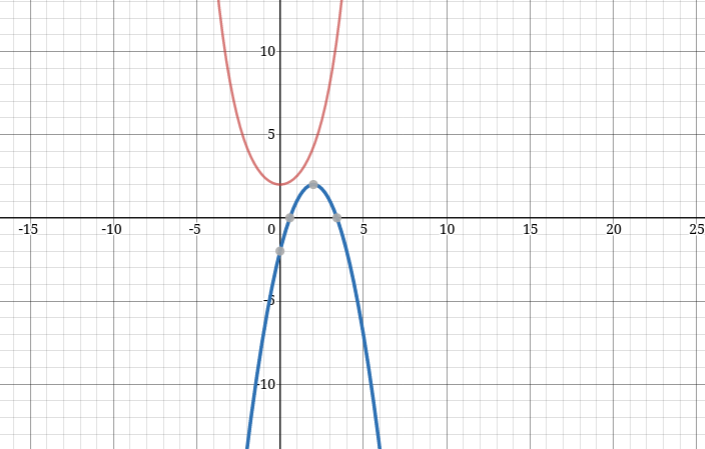Question 4

# The number of real-valued solutions of the equation $$2^{x}+2^{-x}=2-(x-2)^{2}$$ is:

SolutionThe graphs of $$2^{x}+2^{-x} and 2-(x-2)^{2}$$ never intersect. So, number of solutions=0.

Alternate method:

We notice that the minimum value of the term in the LHS will be greater than or equal to 2 {at x=0; LHS = 2}. However, the term in the RHS is less than or equal to 2 {at x=2; RHS = 2}. The values of x at which both the sides become 2 are distinct; hence, there are zero real-valued solutions to the above equation.

### View Video Solution

##### om bikash

1 month, 1 week ago

Hare Krishna hare Krishna Krishna Krishna hare hare
Hare ram hare ram ram ram hare hare

OR